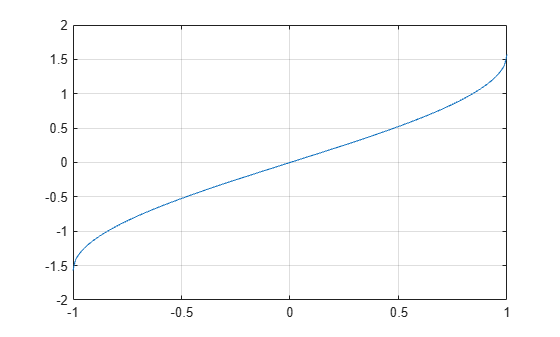# asin

## Syntax

``Y = asin(X)``

## Description

example

````Y = asin(X)` returns the Inverse Sine (sin-1) of the elements of `X` in radians. The function accepts both real and complex inputs. For real values of `X` in the interval [-1, 1], `asin(X)` returns values in the interval [-π/2, π/2].For real values of `X` outside the interval [-1, 1] and for complex values of `X`, `asin(X)` returns complex values. ```

## Examples

collapse all

Find the inverse sine of a value.

`y = asin(1)`
```y = 1.5708 ```

Find the inverse sine of the elements of vector `x`. The `asin` function acts on `x` element-wise.

```x = [0.5i 1+3i -2.2+i]; y = asin(x)```
```y = 1×3 complex 0.0000 + 0.4812i 0.3076 + 1.8642i -1.1091 + 1.5480i ```

Plot the inverse sine function over the intervals $-1\le x\le 1$.

```x = -1:.01:1; plot(x,asin(x)) grid on```## Input Arguments

collapse all

Sine of angle, specified as a scalar, vector, matrix, multidimensional array, table, or timetable. The `asin` operation is element-wise when `X` is nonscalar.

Data Types: `single` | `double` | `table` | `timetable`
Complex Number Support: Yes

collapse all

### Inverse Sine

The inverse sine is defined as

`${\mathrm{sin}}^{-1}\left(z\right)=-i\mathrm{log}\left[iz+{\left(1-{z}^{2}\right)}^{1/2}\right].$`

## Version History

Introduced in R2006a

expand all